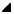# gmp_lib.mpz_divisible_ui_p Method

#### GMP Native Interface for .NET

 gmp_libmpz_divisible_ui_p Method
Return non-zero if n is exactly divisible by d.

Namespace:  Math.Gmp.Native
Assembly:  Math.Gmp.Native (in Math.Gmp.Native.dll) Version: 1.0.0.0 (1.0.0.0)Syntax
```public static int mpz_divisible_ui_p(
mpz_t n,
uint d
)```
```Public Shared Function mpz_divisible_ui_p (
n As mpz_t,
d As UInteger
) As Integer```
```public:
static int mpz_divisible_ui_p(
mpz_t^ n,
unsigned int d
)```
```static member mpz_divisible_ui_p :
n : mpz_t *
d : uint32 -> int
```

#### Parameters

n
Type: Math.Gmp.Nativempz_t
The numerator integer.
d
Type: SystemUInt32
The denominator integer.

#### Return Value

Type: Int32
Non-zero if n is exactly divisible by d.Remarks

n is divisible by d if there exists an integer q satisfying n = q * d. Unlike the other division functions, d = 0 is accepted and following the rule it can be seen that only 0 is considered divisible by 0.Examples
```// Create, initialize, and set the value of x to 10000.
mpz_t x = new mpz_t();
gmp_lib.mpz_init_set_ui(x, 10000U);

// Assert that x is divisible by 5.
Assert.IsTrue(gmp_lib.mpz_divisible_ui_p(x, 5U) > 0);

// Release unmanaged memory allocated for x.
gmp_lib.mpz_clear(x);```
```' Create, initialize, and set the value of x to 10000.
Dim x As New mpz_t()
gmp_lib.mpz_init_set_ui(x, 10000UI)

' Assert that x is divisible by 5.
Assert.IsTrue(gmp_lib.mpz_divisible_ui_p(x, 5UI) > 0)

' Release unmanaged memory allocated for x.
gmp_lib.mpz_clear(x)```

No code example is currently available or this language may not be supported.

No code example is currently available or this language may not be supported.See Also